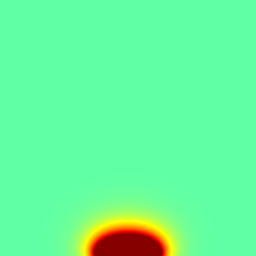# The SAG equation

The SAG equation is a Fick diffusion equation of a specie c \displaystyle \frac{\partial c}{\partial t} =\nabla^2 c which can be solved with the reaction–diffusion solver.

#include "grid/multigrid.h"
#include "run.h"
#include "diffusion.h"

Concentration at time t+dt, t and maximum difference between the two.

scalar c[], cold[];
double errmax;

We will store the statistics on the diffusion solvers in mgd.

mgstats mgd;

A “crystal growth” boundary condition of the form \displaystyle \frac{\partial c}{\partial y} = bi\; c \;\;\mathrm{on}\;\; y=0

is imposed for -1 < x < 1 and y = 0, where bi is a kind of Biot number. This mixed condition can be discretised to second-order accuracy as

(c[] - c[bottom])/Delta = bi*(c[] + c[bottom])/2.;

The rest of the wall is covered with a mask so that no growth occurs i.e. \frac {\partial c}{\partial y} = 0 (a Neumann boundary condition). Combining everything then gives

double bi = 1;
c[bottom] = fabs(x) < 1 ? neumann(0) : c[]*(2. - bi*Delta)/(2. + bi*Delta);

On the top boundary the species concentration is imposed.

c[top] = dirichlet(1);

And there is no flux on the right and left boundaries.

c[right] = neumann(0);
c[left]  = neumann(0);

## Parameters

The domain is the square box [-5:5]\times[0:10].

int main() {
L0 = 10.;
X0 = -L0/2;
N = 256;
errmax = 5.e-3;
run();
}

## Initial conditions

In the absence of a mask (i.e. with the Biot condition along the bottom boundary) an exact stationary solution is

#define cexact(y) ((bi*y + 1)/(bi*L0 + 1))

We use this as initial condition.

event init (i = 0) {
foreach() {
c[] = cexact(y);
cold[] = c[];
}
}

## Time integration

event integration (i++) {

We first set the timestep according to the timing of upcoming events. We choose a maximum timestep of 0.2 which ensures the stability of the reactive terms for this example.

  dt = dtnext (0.2);

We use the diffusion solver to advance the system from t to t+dt.

  mgd = diffusion (c, dt);

If the difference between c and cold is small enough, we stop.

  double dc = change (c, cold);
if (i > 0 && dc < errmax)
return 1; // stop
printf ("%g %g\n", t, dc);
}

## Results

event profiles (i += 10) {
FILE * fpx = fopen("cutx.txt", "w");
FILE * fpy = stderr;

The flux along y is {\partial c}/{\partial y}

  scalar flux[];
foreach()
flux[] = (c[0,0] - c[0,-1])/Delta;

for (double x = -L0/2 ; x < L0/2; x += L0/N) {
double y = x + L0/2;
fprintf (fpx, "%g %g %g\n",
x, interpolate (c, x, 0) , interpolate (flux, x, 0));
fprintf (fpy, "%g %g %g\n",
y, interpolate (c, 0, y) , interpolate (flux, 0, y));
}
fclose(fpx);
}

At the end of the simulation, we create an image of the error field, in PNG format.

event pictures (t = end) {
scalar  dce[];
foreach()
dce[] =  c[] - cexact(y);
output_ppm (dce, file = "c.png", spread = 2, linear = true);
}

We compare the exact and computed solutions for a cross-section at x=0.

bi=1
L0=10
set xlabel "y"
set key left
plot 'log'u 1:2 t 'c' w l, '' u 1:3 t 'dc/dy' w l, \
bi/(bi*L0+1.) t 'bi/(bi L0+1)', \
(bi*x+1)/(bi*L0+1) t'(bi y+1)/(bi*L0+1)'Cross section at x=0 (script)

We also display the concentration flux divided by the reference flux bi/(bi L0+1).

set xlabel "x"
plot './cutx.txt' u 1:($2*(bi*L0+1)) t 'concentration' w l, \ '' u 1:($3*(bi*L0+1)/bi) t 'flux' w l, 1 notCross section at y=0 (script)

And the error field.## Bibliography

• N. Dupuis, J. Décobert, P.-Y. Lagrée, N. Lagay, D. Carpentier, F. Alexandre (2008): “Demonstration of planar thick InP layers by selective MOVPE”. Journal of Crystal Growth issue 23, 15 November 2008, Pages 4795-4798.

• N. Dupuis, J. Décobert, P.-Y. Lagrée , N. Lagay, C. Cuisin, F. Poingt, C. Kazmierski, A. Ramdane, A. Ougazzaden (2008): “Mask pattern interference in AlGaInAs MOVPE Selective Area Growth : experimental and modeling analysis”. Journal of Applied Physics 103, 113113 (2008).